|

# 仿木纹砖 法恩莎仿古砖原木100系列加州橡木评测

典雅的枫林，天姿国色的枫叶，枫之国的问候，从皇室的百叶窗下传出，奢华大气的庭院，深深的几许枫树情怀，从来都是皇家的风范。

本期搜狐焦点家居评测中心为大家带来的是法恩莎原木100系列之加州橡木系列，自然的枫木纹理，清新淡雅却不失庄重典贵，追求一种贵族般的享受，寓豁达的人生情怀与枫林的魅力之中。下面我们先来通过简单的案例展示，看看这款瓷砖的实际使用效果。

法恩莎仿古砖加州橡木不仅可以作为地面砖使用，还可以作为墙面砖使用。同时可以多个空间使用，如书房、卧室、会客厅、雅座、酒店餐厅、高级会所等。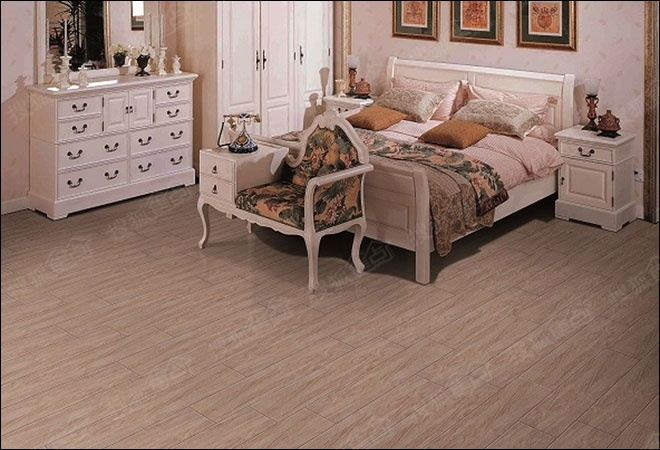法恩莎仿古砖加州橡木实际使用效果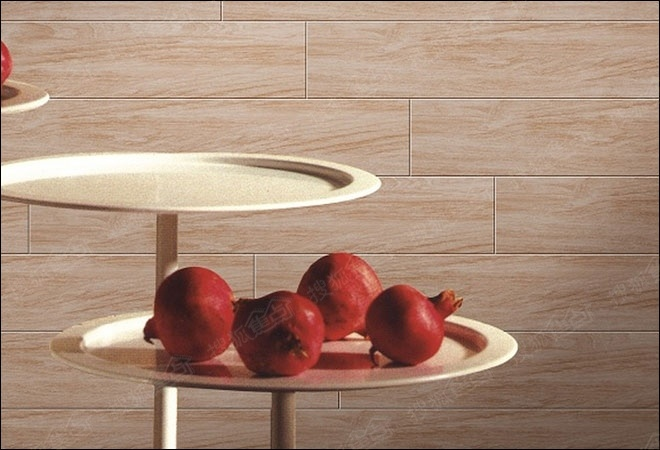法恩莎仿古砖加州橡木实际使用效果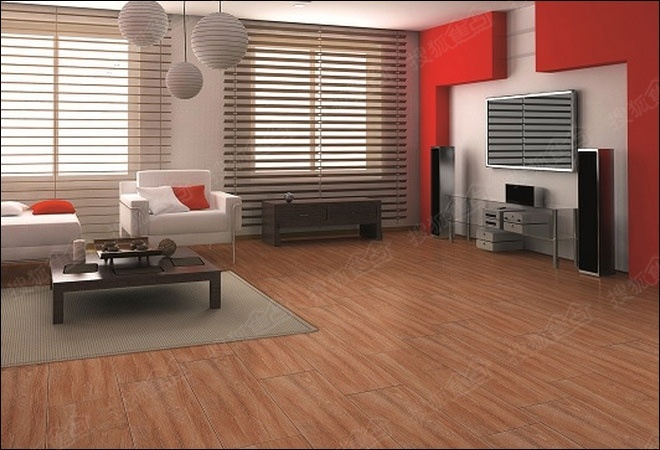法恩莎仿古砖加州橡木实际使用效果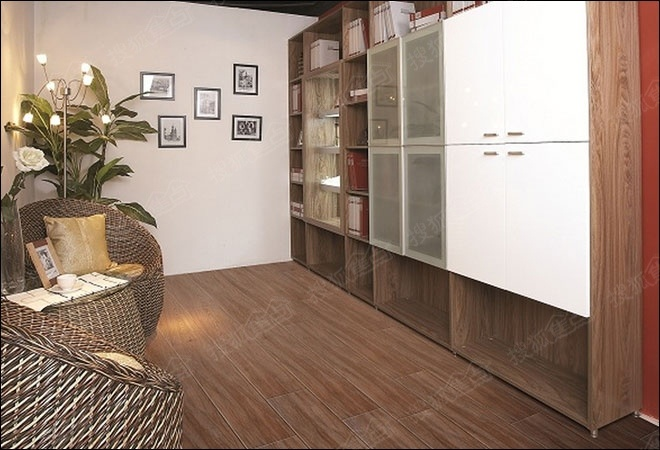法恩莎仿古砖加州橡木实际使用效果

看完了以上案例，是不是想进一步了解这款瓷砖呢？下面跟随我们的评测，一起来认识一下这款瓷砖吧。

加州橡木系列一共包含了8个型号，分别是FCM93531、FCM93532、FCM93534、FCM93538、FCM95531、FCM95532、FCM95534、FCM95538。在类别上，属于仿古砖。在尺寸上，主要有三种选择，分别是900×300mm、900×150mm、900×600mm。颜色用四种颜色，分别是白、黄、红、棕。搜狐焦点评测中心选取了型号为FCM95538的瓷砖。法恩莎仿古砖加州橡木外观

法恩莎仿古砖加州橡木表面纹理为枫木纹理，颜色均匀，纹理自然，彷如实木。900×150mm的尺寸设计，也仿照木地板的尺寸设计，铺贴效果更好！法恩莎仿古砖加州橡木纹理

瓷砖的平整度会直接会影响到拼接后的效果，通过观察法恩莎全抛釉瓷砖戈壁砂岩的侧面直线非常笔直的，没有弯曲，非常平整。仔细观察边角，没有缺边断脚的现象，造工精细。法恩莎仿古砖加州橡木平整度

在法恩莎全抛釉瓷砖戈壁砂岩的背面，印刷上了“faenzh”的品牌标识，确保产品不会被仿制，同时有利于消费者去辨别产品的真伪，这也是法恩莎瓷砖给消费者的信心保证。背面纹理采用的是不规则凸起的纹理搭配，适合铺装后保持瓷砖位置的稳定性。法恩莎仿古砖加州橡木背面纹理

法恩莎仿古砖加州橡木基本信息

品牌：法恩莎

系列：法恩莎原木100

规格：900×150mm

产品型号：FCM95538

评测时间：2014年10月

===============================================

“FAENZA法恩莎”采用意大利先进的生产设备及工艺标准， 秉承“唯美、优雅、艺术”的设计理念，严格执行ISO9001-2004国际质量体系认证、ISO14001-2004环境管理体系认证和环保总局“中国环境标志产品”认证体系，意大利顶级设计师领衔，强大设计师团队创作，产品以高雅别致的外观和高科技含量为一体的内在品质， 体现功能与美感的有机结合，不断致力于为全球消费者提供高品质家居生活的全新享受，引领国际家居空间新潮流。

===============================================

阅读索引：

Page1：法恩莎仿古砖加州橡木简介及外观

Page2：法恩莎仿古砖加州橡木各项测试及总结

·独特而精致 简一大理石瓷砖鹅毛金3代评测

·独家首创 蒙娜丽莎罗马天韵石系列全抛釉瓷砖评测

·做好功课放心买!双十一售后服务条款内容解读X

仿木纹砖，它具有逼真度高；本身具有阻燃，不腐蚀的特点，是绿色、环保型建材。产品使用寿命长，耐磨，无需像木制产品那样周期性地打蜡保养等特征。

下面通过各项测试，让我们一起来看看这款瓷砖的表现效果吧！搜狐焦点家居评测中心将从吸水率、抗污性、防滑性、致密度、耐磨性五项测试进行检验。

【吸水率测试】

评测标准：首先进行的是吸水率测试，吸水率是指石材在标准大气压力下吸水的能力。石材的吸水率是由其中空隙的数量和大小、颗粒相互排列的方式等因素而定。吸水率越小石材越紧密坚硬，石材的吸水率愈大，则其工程性质就愈差。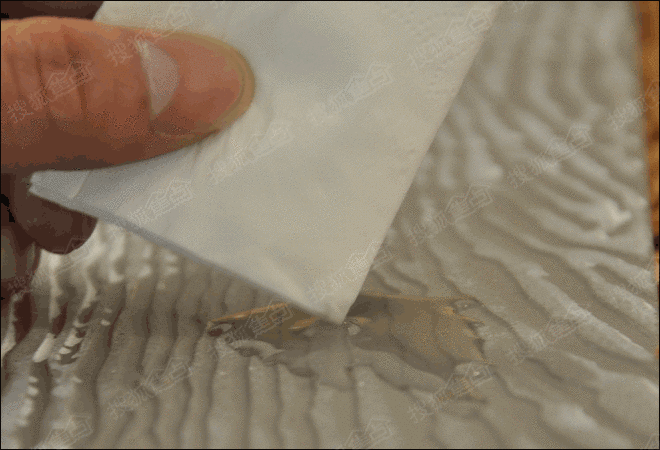法恩莎仿古砖加州橡木吸水率测试

评测结果：搜狐焦点家居首先在瓷砖背面倒上一部分的饮用水液体，经过5分钟的实际观察，砖体没有将水分吸收，液体在背面原封不动。用吸水纸吸去水分，只见吸水纸被快速湿润。最后只留下被水珠润湿过的痕迹，由此表明，这款瓷砖的吸水率是比较低的，证明这款瓷砖的工程性质优秀。

【抗污性测试】

评测标准：地板砖每天都会接触到灰尘等污迹，如果抗污性差，日常的保养与清洁的难度越高，并且影响使用效果与使用体验。油性笔可谓是最顽固的污迹了，如果油性笔也能轻松擦除，则意味着日常生活中的污迹也能轻松擦除。法恩莎仿古砖加州橡木抗污性测试

评测结果：笔者采用油性笔往砖体表面写字，通过实测表明，用纸巾就能将字迹完全清理干净，完全不需要用到洗涤剂，这款产品的防污性良好。

【防滑性测试】

评测标准：如果地板砖滑，防滑性能差，人在上面走动则容易滑倒。因此，地面砖必须具备一定的防滑性能。法恩莎仿古砖加州橡木防滑性测试

评测结果：笔者采用的评测方法是在倾斜30°的瓷砖表面放上装满水的纸杯，观察纸杯是否会下滑。从实际测试来看，纸杯在瓷砖表面能够“站得”很稳，丝毫没有往下滑动的倾向，防滑性能过关。

【致密度测试】

评测标准：砖体的致密度高，则意味着瓷化程度高。瓷化程度高的瓷砖，更加耐用，不易出现断裂等情况。笔者采用的是握拳敲击砖体听声音方式。法恩莎仿古砖加州橡木致密度测试

评测结果：敲击方式需要敲打的部位为瓷砖的中部，砖体发出的声音清脆，没有杂音，表明这款瓷砖瓷化程度高。

【耐磨性测试】

评测标准：耐磨性方面，对于瓷砖来说重要的检测项目，如果耐磨性不过关，使用一段时间以后，瓷砖表面就容易出现很多划痕而影响视觉效果。编辑采用两种方案进行耐磨性评测，一是采用钢丝擦细微摩擦；二是采用螺丝刀用力刮划。法恩莎仿古砖加州橡木耐磨性测试

评测结果：通过实际测试，使用钢丝擦完全没有对法恩莎仿古砖加州橡木造成任何损伤。使用螺丝刀用力刮划表面后，仔细观察也没有发现有对砖体表面造成划痕，哪怕是比较轻微的痕迹也找不到，证明这款瓷砖的耐磨性能优秀。

焦点家居评测总结：

法恩莎仿古砖加州橡木采用精湛的生产制作技术，造工精细，平整度高，拼合效果好，铺贴效果优秀，具有较强的装饰性。不仅可以作为地面砖使用，还可以作为墙面砖使用，同时适用于多个空间。除此之外，法恩莎仿古砖加州橡木还是一款实用性强的仿木纹砖。通过测试，我们看到了这款瓷砖的吸水率低，致密度高、抗污性强、耐磨度高、防滑性强，可以预见日后的使用寿命相对较长，以及易于日常的保养与清洁。

阅读索引：

Page1：法恩莎仿古砖加州橡木简介及外观

Page2：法恩莎仿古砖加州橡木各项测试及总结

·独特而精致 简一大理石瓷砖鹅毛金3代评测

·独家首创 蒙娜丽莎罗马天韵石系列全抛釉瓷砖评测

·做好功课放心买!双十一售后服务条款内容解读

`声明：本文由入驻焦点开放平台的作者撰写，除焦点官方账号外，观点仅代表作者本人，不代表焦点立场错误信息举报电话： 400-099-0099，邮箱：jubao@vip.sohu.com，或点此进行意见反馈，或点此进行举报投诉。`A B C D E F G H J K L M N P Q R S T W X Y Z
A - B - C - D - E
• A
• 鞍山
• 安庆
• 安阳
• 安顺
• 安康
• 澳门
• B
• 北京
• 保定
• 包头
• 巴彦淖尔
• 本溪
• 蚌埠
• 亳州
• 滨州
• 北海
• 百色
• 巴中
• 毕节
• 保山
• 宝鸡
• 白银
• 巴州
• C
• 承德
• 沧州
• 长治
• 赤峰
• 朝阳
• 长春
• 常州
• 滁州
• 池州
• 长沙
• 常德
• 郴州
• 潮州
• 崇左
• 重庆
• 成都
• 楚雄
• 昌都
• 慈溪
• 常熟
• D
• 大同
• 大连
• 丹东
• 大庆
• 东营
• 德州
• 东莞
• 德阳
• 达州
• 大理
• 德宏
• 定西
• 儋州
• 东平
• E
• 鄂尔多斯
• 鄂州
• 恩施
F - G - H - I - J
• F
• 抚顺
• 阜新
• 阜阳
• 福州
• 抚州
• 佛山
• 防城港
• G
• 赣州
• 广州
• 桂林
• 贵港
• 广元
• 广安
• 贵阳
• 固原
• H
• 邯郸
• 衡水
• 呼和浩特
• 呼伦贝尔
• 葫芦岛
• 哈尔滨
• 黑河
• 淮安
• 杭州
• 湖州
• 合肥
• 淮南
• 淮北
• 黄山
• 菏泽
• 鹤壁
• 黄石
• 黄冈
• 衡阳
• 怀化
• 惠州
• 河源
• 贺州
• 河池
• 海口
• 红河
• 汉中
• 海东
• I
• J
• 晋中
• 锦州
• 吉林
• 鸡西
• 佳木斯
• 嘉兴
• 金华
• 景德镇
• 九江
• 吉安
• 济南
• 济宁
• 焦作
• 荆门
• 荆州
• 江门
• 揭阳
• 金昌
• 酒泉
• 嘉峪关
K - L - M - N - P
• K
• 开封
• 昆明
• 昆山
• L
• 廊坊
• 临汾
• 辽阳
• 连云港
• 丽水
• 六安
• 龙岩
• 莱芜
• 临沂
• 聊城
• 洛阳
• 漯河
• 娄底
• 柳州
• 来宾
• 泸州
• 乐山
• 六盘水
• 丽江
• 临沧
• 拉萨
• 林芝
• 兰州
• 陇南
• M
• 牡丹江
• 马鞍山
• 茂名
• 梅州
• 绵阳
• 眉山
• N
• 南京
• 南通
• 宁波
• 南平
• 宁德
• 南昌
• 南阳
• 南宁
• 内江
• 南充
• P
• 盘锦
• 莆田
• 平顶山
• 濮阳
• 攀枝花
• 普洱
• 平凉
Q - R - S - T - W
• Q
• 秦皇岛
• 齐齐哈尔
• 衢州
• 泉州
• 青岛
• 清远
• 钦州
• 黔南
• 曲靖
• 庆阳
• R
• 日照
• 日喀则
• S
• 石家庄
• 沈阳
• 双鸭山
• 绥化
• 上海
• 苏州
• 宿迁
• 绍兴
• 宿州
• 三明
• 上饶
• 三门峡
• 商丘
• 十堰
• 随州
• 邵阳
• 韶关
• 深圳
• 汕头
• 汕尾
• 三亚
• 三沙
• 遂宁
• 山南
• 商洛
• 石嘴山
• T
• 天津
• 唐山
• 太原
• 通辽
• 铁岭
• 泰州
• 台州
• 铜陵
• 泰安
• 铜仁
• 铜川
• 天水
• 天门
• W
• 乌海
• 乌兰察布
• 无锡
• 温州
• 芜湖
• 潍坊
• 威海
• 武汉
• 梧州
• 渭南
• 武威
• 吴忠
• 乌鲁木齐
X - Y - Z
• X
• 邢台
• 徐州
• 宣城
• 厦门
• 新乡
• 许昌
• 信阳
• 襄阳
• 孝感
• 咸宁
• 湘潭
• 湘西
• 西双版纳
• 西安
• 咸阳
• 西宁
• 仙桃
• 西昌
• Y
• 运城
• 营口
• 盐城
• 扬州
• 鹰潭
• 宜春
• 烟台
• 宜昌
• 岳阳
• 益阳
• 永州
• 阳江
• 云浮
• 玉林
• 宜宾
• 雅安
• 玉溪
• 延安
• 榆林
• 银川
• Z
• 张家口
• 镇江
• 舟山
• 漳州
• 淄博
• 枣庄
• 郑州
• 周口
• 驻马店
• 株洲
• 张家界
• 珠海
• 湛江
• 肇庆
• 中山
• 自贡
• 资阳
• 遵义
• 昭通
• 张掖
• 中卫

1室1厅1厨1卫1阳台

1
2
3
4
5

0
1
2

1

1

0
1
2
3报名成功，资料已提交审核A B C D E F G H J K L M N P Q R S T W X Y Z
A - B - C - D - E
• A
• 鞍山
• 安庆
• 安阳
• 安顺
• 安康
• 澳门
• B
• 北京
• 保定
• 包头
• 巴彦淖尔
• 本溪
• 蚌埠
• 亳州
• 滨州
• 北海
• 百色
• 巴中
• 毕节
• 保山
• 宝鸡
• 白银
• 巴州
• C
• 承德
• 沧州
• 长治
• 赤峰
• 朝阳
• 长春
• 常州
• 滁州
• 池州
• 长沙
• 常德
• 郴州
• 潮州
• 崇左
• 重庆
• 成都
• 楚雄
• 昌都
• 慈溪
• 常熟
• D
• 大同
• 大连
• 丹东
• 大庆
• 东营
• 德州
• 东莞
• 德阳
• 达州
• 大理
• 德宏
• 定西
• 儋州
• 东平
• E
• 鄂尔多斯
• 鄂州
• 恩施
F - G - H - I - J
• F
• 抚顺
• 阜新
• 阜阳
• 福州
• 抚州
• 佛山
• 防城港
• G
• 赣州
• 广州
• 桂林
• 贵港
• 广元
• 广安
• 贵阳
• 固原
• H
• 邯郸
• 衡水
• 呼和浩特
• 呼伦贝尔
• 葫芦岛
• 哈尔滨
• 黑河
• 淮安
• 杭州
• 湖州
• 合肥
• 淮南
• 淮北
• 黄山
• 菏泽
• 鹤壁
• 黄石
• 黄冈
• 衡阳
• 怀化
• 惠州
• 河源
• 贺州
• 河池
• 海口
• 红河
• 汉中
• 海东
• I
• J
• 晋中
• 锦州
• 吉林
• 鸡西
• 佳木斯
• 嘉兴
• 金华
• 景德镇
• 九江
• 吉安
• 济南
• 济宁
• 焦作
• 荆门
• 荆州
• 江门
• 揭阳
• 金昌
• 酒泉
• 嘉峪关
K - L - M - N - P
• K
• 开封
• 昆明
• 昆山
• L
• 廊坊
• 临汾
• 辽阳
• 连云港
• 丽水
• 六安
• 龙岩
• 莱芜
• 临沂
• 聊城
• 洛阳
• 漯河
• 娄底
• 柳州
• 来宾
• 泸州
• 乐山
• 六盘水
• 丽江
• 临沧
• 拉萨
• 林芝
• 兰州
• 陇南
• M
• 牡丹江
• 马鞍山
• 茂名
• 梅州
• 绵阳
• 眉山
• N
• 南京
• 南通
• 宁波
• 南平
• 宁德
• 南昌
• 南阳
• 南宁
• 内江
• 南充
• P
• 盘锦
• 莆田
• 平顶山
• 濮阳
• 攀枝花
• 普洱
• 平凉
Q - R - S - T - W
• Q
• 秦皇岛
• 齐齐哈尔
• 衢州
• 泉州
• 青岛
• 清远
• 钦州
• 黔南
• 曲靖
• 庆阳
• R
• 日照
• 日喀则
• S
• 石家庄
• 沈阳
• 双鸭山
• 绥化
• 上海
• 苏州
• 宿迁
• 绍兴
• 宿州
• 三明
• 上饶
• 三门峡
• 商丘
• 十堰
• 随州
• 邵阳
• 韶关
• 深圳
• 汕头
• 汕尾
• 三亚
• 三沙
• 遂宁
• 山南
• 商洛
• 石嘴山
• T
• 天津
• 唐山
• 太原
• 通辽
• 铁岭
• 泰州
• 台州
• 铜陵
• 泰安
• 铜仁
• 铜川
• 天水
• 天门
• W
• 乌海
• 乌兰察布
• 无锡
• 温州
• 芜湖
• 潍坊
• 威海
• 武汉
• 梧州
• 渭南
• 武威
• 吴忠
• 乌鲁木齐
X - Y - Z
• X
• 邢台
• 徐州
• 宣城
• 厦门
• 新乡
• 许昌
• 信阳
• 襄阳
• 孝感
• 咸宁
• 湘潭
• 湘西
• 西双版纳
• 西安
• 咸阳
• 西宁
• 仙桃
• 西昌
• Y
• 运城
• 营口
• 盐城
• 扬州
• 鹰潭
• 宜春
• 烟台
• 宜昌
• 岳阳
• 益阳
• 永州
• 阳江
• 云浮
• 玉林
• 宜宾
• 雅安
• 玉溪
• 延安
• 榆林
• 银川
• Z
• 张家口
• 镇江
• 舟山
• 漳州
• 淄博
• 枣庄
• 郑州
• 周口
• 驻马店
• 株洲
• 张家界
• 珠海
• 湛江
• 肇庆
• 中山
• 自贡
• 资阳
• 遵义
• 昭通
• 张掖
• 中卫• 手机• 分享
• 设计
免费设计
• 计算器
装修计算器
• 入驻
合作入驻
• 联系
联系我们
• 置顶
返回顶部